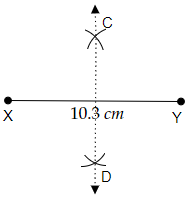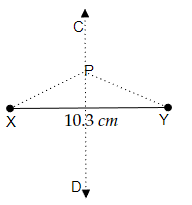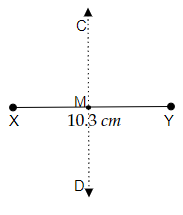# Draw the perpendicular bisector of $\overline{X Y}$ whose length is $10.3 \mathrm{~cm}$.(a) Take any point $\mathrm{P}$ on the bisector drawn. Examine whether $\mathrm{PX}=\mathrm{PY}$.(b) If $\mathrm{M}$ is the mid point of $\overline{\mathrm{XY}}$, what can you say about the lengths $\mathrm{MX}$ and $\mathrm{XY}$ ?

To do:

We have to draw the perpendicular bisector of $\overline{XY}$ whose length is $10.3\ cm$ and answer the given questions.

Solution:Steps of construction:

(i) Let us draw a line segment $\overline{XY}$ of length $10.3\ cm$ .

(ii) Now, with compasses take a measure greater than half of the length $\overline{XY}$

(iii) Now, by pointing the pointer of the compasses at point X and point Y respectively. let's draw two arcs above the line segment $\overline{XY}$ cutting each other and point it as C.

(iv) Similarly, by pointing the pointer of the compasses at point X and point Y respectively. let's draw another two arcs below the line segment $\overline{XY}$ cutting each other and point it as D.

(iv) Then, let's draw a line segment joining point C and point D.

(v) Therefore, the perpendicular bisector of the line segment $\overline{XY}$ is formed.

(a) Let us take any point P on the bisector CD.Since $\overline{CD}$ is the perpendicular bisector of $\overline{XY}$ any point lying on $\overline{CD}$ will be at the same distance from point X and point Y of line segment $\overline{XY}$.

Therefore,

We may measure the length of $PX = PY$.

(b) As M is the midpoint of $\overline{XY}$. The perpendicular bisector $\overline{CD}$ passes through point M.Therefore,

The length of $\overline{XY}$ is twice $\overline{MX}$.

That is $\overline{MX}$ is $\frac{1}{2}\overline{XY}$.

Updated on: 10-Oct-2022

21 Views